# RD Sharma Solutions for Class 6 Maths Chapter 2: Playing with Numbers Exercise 2.8

## RD Sharma Solutions for Class 6 Maths Exercise 2.8 PDF

RD Sharma Solutions is a vital resource which can be used by students to ace the exam. The set of faculty at BYJU’S having vast experience in the education industry prepare solutions after conducting research on each concept. The solutions are explained in a simple language which matches the understanding ability of the students. PDF of solutions is available for all the chapters based on CBSE guidelines and can be used as a reference guide. RD Sharma Solutions for Class 6 Maths Chapter 2 Playing with Numbers Exercise 2.8 are provided here.

## RD Sharma Solutions for Class 6 Maths Chapter 2: Playing with Numbers Exercise 2.8 Download PDF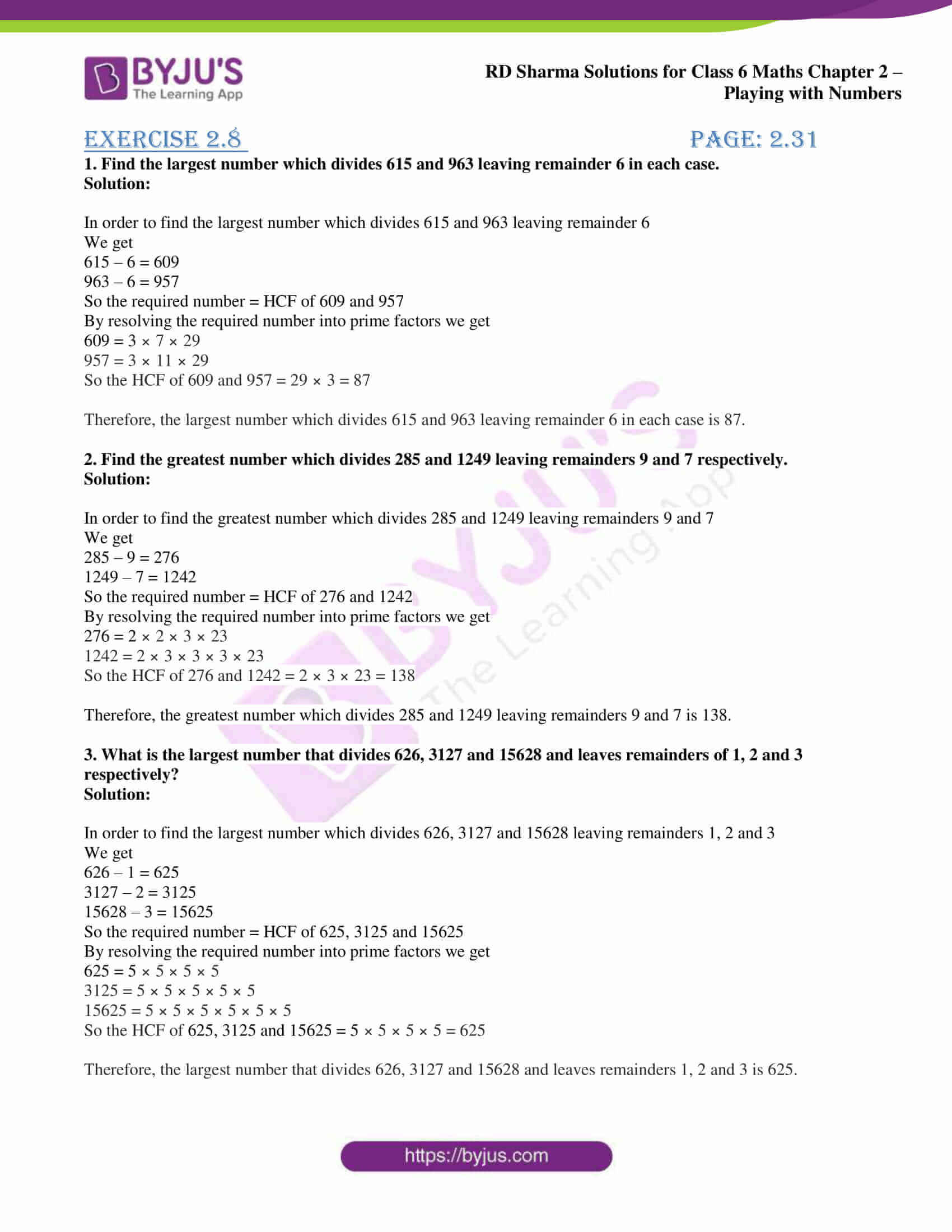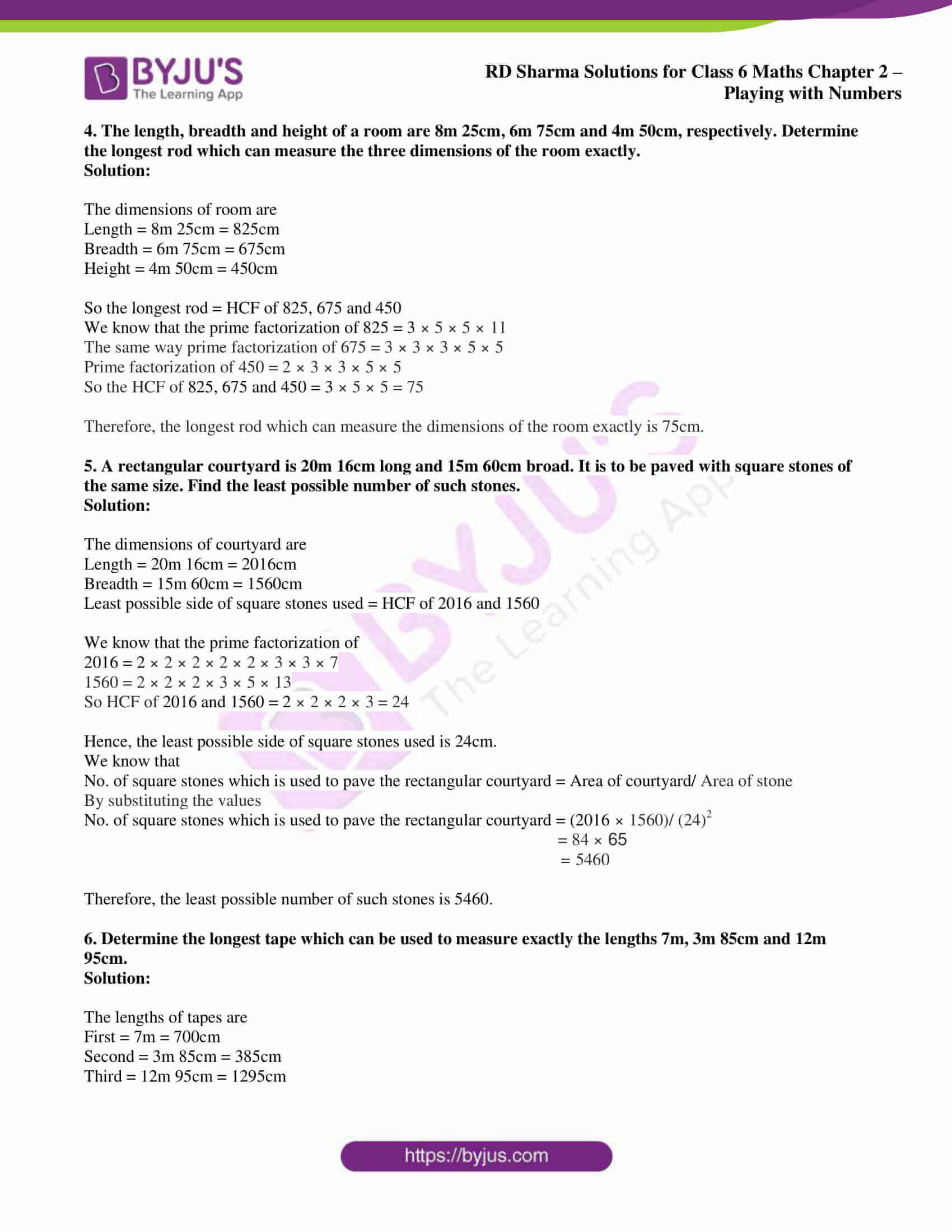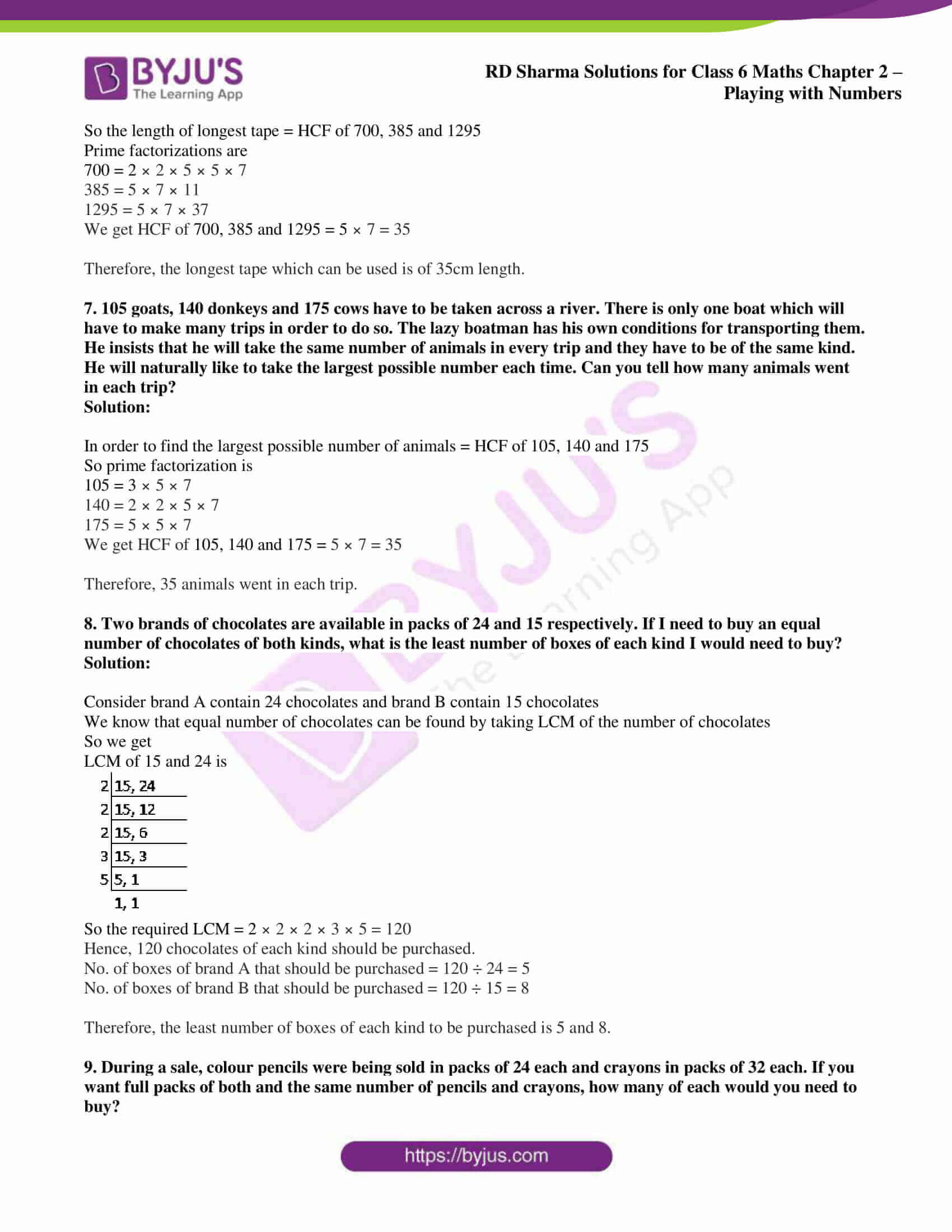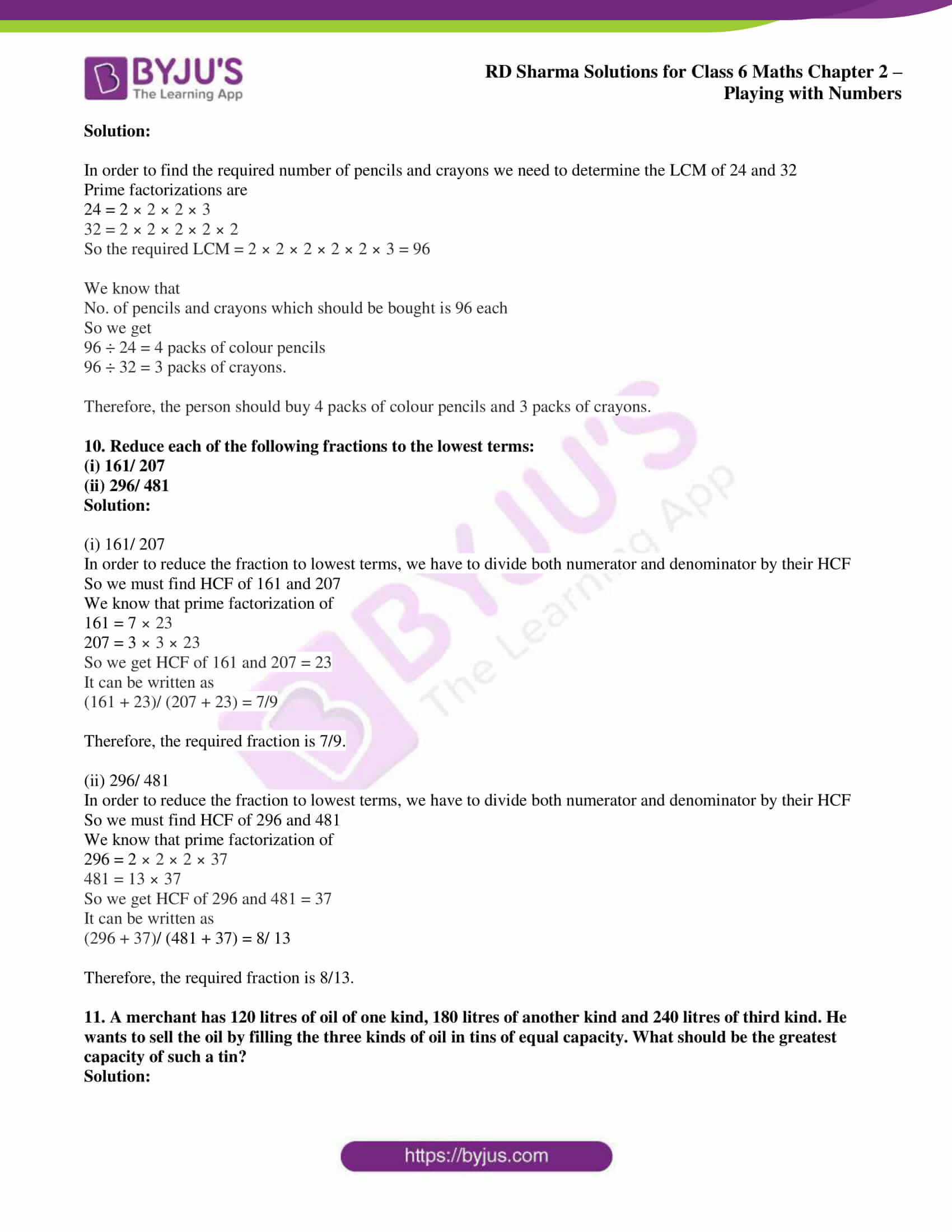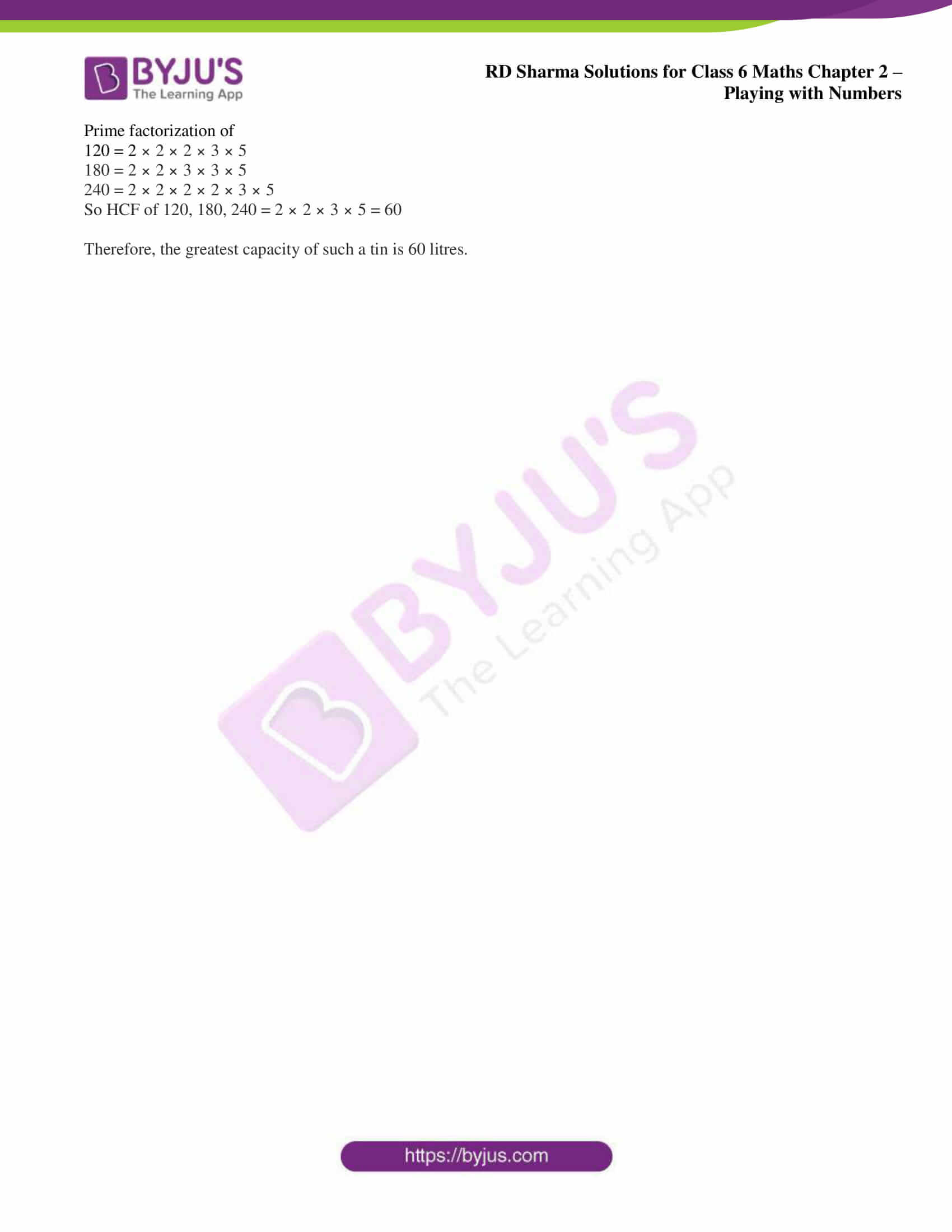## Access answers to Maths RD Sharma Solutions for Class 6 Chapter 2: Playing with Numbers Exercise 2.8

1. Find the largest number which divides 615 and 963 leaving remainder 6 in each case.

Solution:

In order to find the largest number which divides 615 and 963 leaving remainder 6

We get

615 – 6 = 609

963 – 6 = 957

So the required number = HCF of 609 and 957

By resolving the required number into prime factors we get

609 = 3 × 7 × 29

957 = 3 × 11 × 29

So the HCF of 609 and 957 = 29 × 3 = 87

Therefore, the largest number which divides 615 and 963 leaving remainder 6 in each case is 87.

2. Find the greatest number which divides 285 and 1249 leaving remainders 9 and 7 respectively.

Solution:

In order to find the greatest number which divides 285 and 1249 leaving remainders 9 and 7

We get

285 – 9 = 276

1249 – 7 = 1242

So the required number = HCF of 276 and 1242

By resolving the required number into prime factors we get

276 = 2 × 2 × 3 × 23

1242 = 2 × 3 × 3 × 3 × 23

So the HCF of 276 and 1242 = 2 × 3 × 23 = 138

Therefore, the greatest number which divides 285 and 1249 leaving remainders 9 and 7 is 138.

3. What is the largest number that divides 626, 3127 and 15628 and leaves remainders of 1, 2 and 3 respectively?

Solution:

In order to find the largest number which divides 626, 3127 and 15628 leaving remainders 1, 2 and 3

We get

626 – 1 = 625

3127 – 2 = 3125

15628 – 3 = 15625

So the required number = HCF of 625, 3125 and 15625

By resolving the required number into prime factors we get

625 = 5 × 5 × 5 × 5

3125 = 5 × 5 × 5 × 5 × 5

15625 = 5 × 5 × 5 × 5 × 5 × 5

So the HCF of 625, 3125 and 15625 = 5 × 5 × 5 × 5 = 625

Therefore, the largest number that divides 626, 3127 and 15628 and leaves remainders 1, 2 and 3 is 625.

4. The length, breadth and height of a room are 8m 25cm, 6m 75cm and 4m 50cm, respectively. Determine the longest rod which can measure the three dimensions of the room exactly.

Solution:

The dimensions of room are

Length = 8m 25cm = 825cm

Breadth = 6m 75cm = 675cm

Height = 4m 50cm = 450cm

So the longest rod = HCF of 825, 675 and 450

We know that the prime factorization of 825 = 3 × 5 × 5 × 11

The same way prime factorization of 675 = 3 × 3 × 3 × 5 × 5

Prime factorization of 450 = 2 × 3 × 3 × 5 × 5

So the HCF of 825, 675 and 450 = 3 × 5 × 5 = 75

Therefore, the longest rod which can measure the dimensions of the room exactly is 75cm.

5. A rectangular courtyard is 20m 16cm long and 15m 60cm broad. It is to be paved with square stones of the same size. Find the least possible number of such stones.

Solution:

The dimensions of courtyard are

Length = 20m 16cm = 2016cm

Breadth = 15m 60cm = 1560cm

Least possible side of square stones used = HCF of 2016 and 1560

We know that the prime factorization of

2016 = 2 × 2 × 2 × 2 × 2 × 3 × 3 × 7

1560 = 2 × 2 × 2 × 3 × 5 × 13

So HCF of 2016 and 1560 = 2 × 2 × 2 × 3 = 24

Hence, the least possible side of square stones used is 24cm.

We know that

No. of square stones which is used to pave the rectangular courtyard = Area of courtyard/ Area of stone

By substituting the values

No. of square stones which is used to pave the rectangular courtyard = (2016 × 1560)/ (24)2

= 84 × 65

= 5460

Therefore, the least possible number of such stones is 5460.

6. Determine the longest tape which can be used to measure exactly the lengths 7m, 3m 85cm and 12m 95cm.

Solution:

The lengths of tapes are

First = 7m = 700cm

Second = 3m 85cm = 385cm

Third = 12m 95cm = 1295cm

So the length of longest tape = HCF of 700, 385 and 1295

Prime factorizations are

700 = 2 × 2 × 5 × 5 × 7

385 = 5 × 7 × 11

1295 = 5 × 7 × 37

We get HCF of 700, 385 and 1295 = 5 × 7 = 35

Therefore, the longest tape which can be used is of 35cm length.

7. 105 goats, 140 donkeys and 175 cows have to be taken across a river. There is only one boat which will have to make many trips in order to do so. The lazy boatman has his own conditions for transporting them. He insists that he will take the same number of animals in every trip and they have to be of the same kind. He will naturally like to take the largest possible number each time. Can you tell how many animals went in each trip?

Solution:

In order to find the largest possible number of animals = HCF of 105, 140 and 175

So prime factorization is

105 = 3 × 5 × 7

140 = 2 × 2 × 5 × 7

175 = 5 × 5 × 7

We get HCF of 105, 140 and 175 = 5 × 7 = 35

Therefore, 35 animals went in each trip.

8. Two brands of chocolates are available in packs of 24 and 15 respectively. If I need to buy an equal number of chocolates of both kinds, what is the least number of boxes of each kind I would need to buy?

Solution:

Consider brand A contain 24 chocolates and brand B contain 15 chocolates

We know that equal number of chocolates can be found by taking LCM of the number of chocolates

So we get

LCM of 15 and 24 is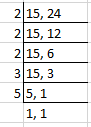So the required LCM = 2 × 2 × 2 × 3 × 5 = 120

Hence, 120 chocolates of each kind should be purchased.

No. of boxes of brand A that should be purchased = 120 ÷ 24 = 5

No. of boxes of brand B that should be purchased = 120 ÷ 15 = 8

Therefore, the least number of boxes of each kind to be purchased is 5 and 8.

9. During a sale, colour pencils were being sold in packs of 24 each and crayons in packs of 32 each. If you want full packs of both and the same number of pencils and crayons, how many of each would you need to buy?

Solution:

In order to find the required number of pencils and crayons we need to determine the LCM of 24 and 32

Prime factorizations are

24 = 2 × 2 × 2 × 3

32 = 2 × 2 × 2 × 2 × 2

So the required LCM = 2 × 2 × 2 × 2 × 2 × 3 = 96

We know that

No. of pencils and crayons which should be bought is 96 each

So we get

96 ÷ 24 = 4 packs of colour pencils

96 ÷ 32 = 3 packs of crayons.

Therefore, the person should buy 4 packs of colour pencils and 3 packs of crayons.

10. Reduce each of the following fractions to the lowest terms:

(i) 161/ 207

(ii) 296/ 481

Solution:

(i) 161/ 207

In order to reduce the fraction to lowest terms, we have to divide both numerator and denominator by their HCF

So we must find HCF of 161 and 207

We know that prime factorization of

161 = 7 × 23

207 = 3 × 3 × 23

So we get HCF of 161 and 207 = 23

It can be written as

(161 + 23)/ (207 + 23) = 7/9

Therefore, the required fraction is 7/9.

(ii) 296/ 481

In order to reduce the fraction to lowest terms, we have to divide both numerator and denominator by their HCF

So we must find HCF of 296 and 481

We know that prime factorization of

296 = 2 × 2 × 2 × 37

481 = 13 × 37

So we get HCF of 296 and 481 = 37

It can be written as

(296 + 37)/ (481 + 37) = 8/ 13

Therefore, the required fraction is 8/13.

11. A merchant has 120 litres of oil of one kind, 180 litres of another kind and 240 litres of third kind. He wants to sell the oil by filling the three kinds of oil in tins of equal capacity. What should be the greatest capacity of such a tin?

Solution:

Prime factorization of

120 = 2 × 2 × 2 × 3 × 5

180 = 2 × 2 × 3 × 3 × 5

240 = 2 × 2 × 2 × 2 × 3 × 5

So HCF of 120, 180, 240 = 2 × 2 × 3 × 5 = 60

Therefore, the greatest capacity of such a tin is 60 litres.

### RD Sharma Solutions for Class 6 Maths Chapter 2 – Playing with Numbers Exercise 2.8

RD Sharma Solutions Class 6 Maths Chapter 2 Playing with Numbers Exercise 2.8 provides the students with knowledge about the applications of HCF in daily life.

### Key features of RD Sharma Solutions for Class 6 Maths Chapter 2: Playing with Numbers Exercise 2.8

• RD Sharma Solutions provides a clear idea about the concepts and important formulas which are covered in each exercise.
• The solutions are prepared in a comprehensive manner in order to help students cover the entire syllabus thoroughly.
• Solving the examples and exercise wise problems help students understand the types of questions that would appear in the exam.
• Practising huge number of problems mainly improves speed of solving and accuracy which is important from the exam point of view.